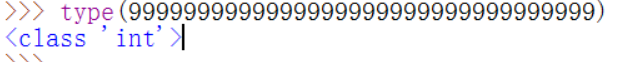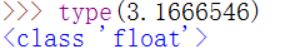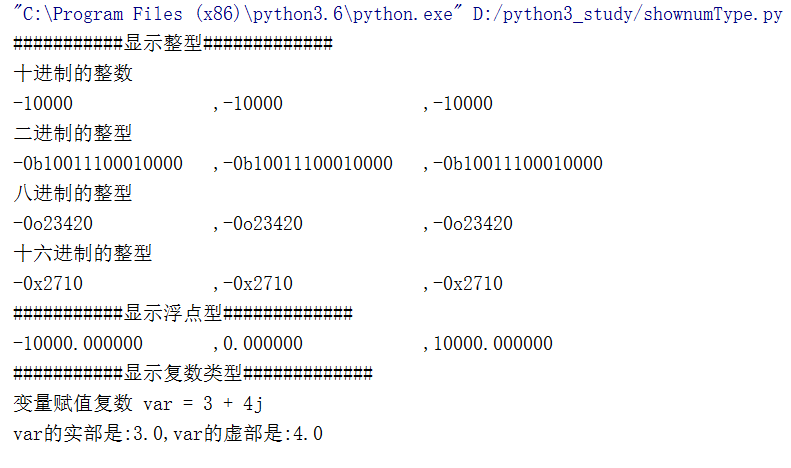## Python变量类型

python3支持3种不同的数值类型

1.int类型

有符号整数,就是C语言中所指的整型,也就是数学中的整数,它的大小与安装的解释器的位数有关

查看当前系统下的Int最大值

打开windows下的idle编辑器(安装完python3后自带)

python3-windows安装方法

输入代码:

```import sys
print(sys.maxsize)```

执行结果如下与别的语言可能有点不同,python给变量赋值时不需要预先声明变量类型

在python2中还会有长整数,超过int类型的整数默认转换Long,一般来说int足够用了，但是在python3中没有长整型，只有int整型

输入代码：

`type(9999999999999999999999999999999999)`

运行结果：2.Float类型(浮点型)

浮点型实数，基本和C语言的浮点型一致，也就是数学中带小数点的数，不包括无限小数，不区分精度，只要是带小数点的数都可以看作浮点型

输入代码

`type(3.1666546)`

运行结果：3.Complex类型

复数，在C语言中是需要自定义的一个数据类型，在python中把它单独列出作为基本数据类型，复数包含一个有序对，表示为a+bj，其中，a是实部，b是复数的虚部

用一个简单的程序shownumType.py，来显示python的数字类型

在Pycharm中创建shownumType.py

代码如下:

```# coding: utf-8
__author__ = 'www.py3study.com'
class shownumType(object):
def __init__(self):
self.showInt()
self.showFloat()
self.showComplex()

#int类型
def showInt(self):
print(u'###########显示整型#############')
print(u'十进制的整数')
print('{0:<20d},{0:<20d},{0:<20d}'.format(-10000, 0, 10000))
print(u'二进制的整型')
print('{0:<20},{0:<20},{0:<20}'.format(bin(-10000),bin(0),bin(10000)))
print(u'八进制的整型')
print('{0:<20},{0:<20},{0:<20}'.format(oct(-10000),oct(0),oct(10000)))
print(u'十六进制的整型')
print('{0:<20},{0:<20},{0:<20}'.format(hex(-10000), hex(0), hex(10000)))

#Float类型
def showFloat(self):
print(u'###########显示浮点型#############')
print('{:<20f},{:<20f},{:<20f}'.format(-10000, 0, 10000))

#Complex复数类型
def showComplex(self):
print(u'###########显示复数类型#############')
print(u'变量赋值复数 var = 3 + 4j')
var = 3 + 4j
print(u'var的实部是:{},var的虚部是:{}'.format(var.real,var.imag))

if __name__ == '__main__':
showNum = shownumType()```

运行结果如下:Ps:注释

shownumType()以C++风格写的程序,展现如何标准输出各种基本数据类型

里面引用了类class,函数def,还有格式化format方法